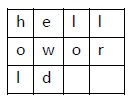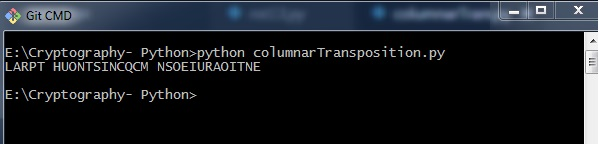# Transposition Cipher

Transposition Cipher is a cryptographic algorithm where the order of alphabets in the plaintext is rearranged to form a cipher text. In this process, the actual plain text alphabets are not included.

## Example

A simple example for a transposition cipher is columnar transposition cipher where each character in the plain text is written horizontally with specified alphabet width. The cipher is written vertically, which creates an entirely different cipher text.

Consider the plain text hello world, and let us apply the simple columnar transposition technique as shown belowThe plain text characters are placed horizontally and the cipher text is created with vertical format as : holewdlo lr. Now, the receiver has to use the same table to decrypt the cipher text to plain text.

## Code

The following program code demonstrates the basic implementation of columnar transposition technique −

def split_len(seq, length):
return [seq[i:i + length] for i in range(0, len(seq), length)]
def encode(key, plaintext):
order = {
int(val): num for num, val in enumerate(key)
}
ciphertext = ''

for index in sorted(order.keys()):
for part in split_len(plaintext, len(key)):
try:ciphertext += part[order[index]]
except IndexError:
continue
return ciphertext
print(encode('3214', 'HELLO'))


### Explanation

• Using the function split_len(), we can split the plain text characters, which can be placed in columnar or row format.

• encode method helps to create cipher text with key specifying the number of columns and prints the cipher text by reading characters through each column.

## Output

The program code for the basic implementation of columnar transposition technique gives the following output −Note − Cryptanalysts observed a significant improvement in crypto security when transposition technique is performed. They also noted that re-encrypting the cipher text using same transposition cipher creates better security.